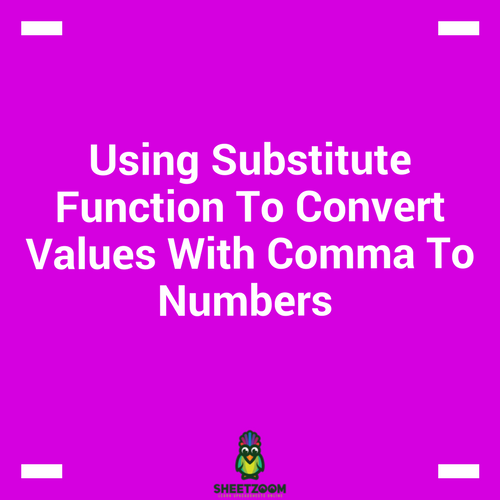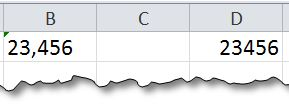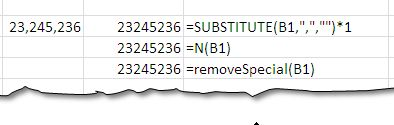# Using Substitute Function To Convert Values With Comma To NumbersWe encounter this problem of numbers behaving as text with commas when we import data from some other software in to excel sheet.  The problem starts with the indication that numbers starts aligning to the left like text and they either have extra spaces or have imprint able characters that do not appear clearly but make numbers behave in an unusual manner.  In this short tutorial, will show you how to make such numbers actually work like numbers!

Consider a Number like this one: 12,645,990Approach # 1 – Substituting commas with a Formula:

Excel has a built in function called SUBSTITUTE() that we use to substitute values in a text. The SUBSTITUTE() has following syntax:

SUBSTITUTE(text, old_text, new_text, [instance_num])

Text is the Text we want to remove the commas from, old text is the text we want to remove for our case this is comma, instance_num is optional, if you specify, the specific instance will be removed, and otherwise all the instances will be removed.

Solution:

The following formula works a treat when substituting for commas

=SUBSTITUTE(B1,",","")*1

Where B1 is the cell in which we have the text and we want to eliminate comma “,”. The returned result is text hence we multiply it with 1 to get a numeric value.

Approach # 2 – Extracting numeric value with N():

N() is another function present under math function section. The function takes following syntax:

N(value)

i.e. it only takes the value and converts it to number.

Approach # 3 – Using VBA to remove characters:

The following vba code using a list marked as RemoveChars that are removed from the string under consideration.

Function removeSpecial(sInput As String) As String

Dim RemoveChars As String

Dim i As Long

RemoveChars = "'" 'This is list

For i = 1 To Len(RemoveChars)

sInput = Replace\$(sInput, Mid\$(RemoveChars, i, 1), "") 'this will remove spaces

Next

removeSpecial = sInput

End FunctionThis function needs to be put in the module in the worksheet or simply copy this to your personal.xlsb to access it from any of the sheet.